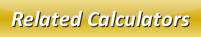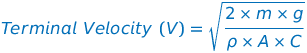# Terminal Velocity Calculator

Terminal velocity is the velocity of a falling object while sum of buoyancy and drag force equals gravity's downward force that is acting upon the given object.

We can calculate the terminal velocity with the help of this below formula:where,
V = Terminal Velocity [m/s]
m = Mass of the object [kg]
g = Acceleration due to gravity [9.8 m/s2]
ρ = Density [kg/m3]
A = Projected area [m2]
C = Drag Coefficient

In the below online terminal velocity calculator, enter the above given parameters into the input boxes and then click calculate button to get the output.

 Mass of the object (m): [kg] Acceleration Due to Gravity (g): [m/s2] Density (ρ): [kg/m3] Projected Area (A): [m2] Drag Coefficient (C): Terminal Velocity (V): [m/s]

Latest Calculator Release

Average Acceleration Calculator

Average acceleration is the object's change in speed for a specific given time period. ...

Free Fall Calculator

When an object falls into the ground due to planet's own gravitational force is known a...

Torque Calculator

Torque is nothing but a rotational force. In other words, the amount of force applied t...

Average Force Calculator

Average force can be explained as the amount of force exerted by the body moving at giv...

Angular Displacement Calculator

Angular displacement is the angle at which an object moves on a circular path. It is de...